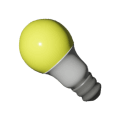Measuring electricity use | BCHydro Power Smart for Schools
Energy

# Measuring electricity use

Calculate the electricity used in a typical household and learn about watts, kilowatts and kilowatt-hours.9Duration
40 minsType
Class discussion

## Overview

Using practical examples of the appliances and objects found in every home, your students perform basic calculations to reveal how much electricity they use.

### Instructions

#### What you'll need

1. Introduce your students to the terms “watts” and “kilowatts” by watching the "How do we measure electricity" video. These are units used when measuring electrical power.
• A watt is the rate that an appliance or device uses energy: 1 watt = 1 joule/second.
• The higher the wattage, the more power the device or appliance uses.
• A kilowatt is 1,000 watts.
2. Remind your class that energy is the ability to do work. Electricity is a source of power that can do work for us. To determine how much electrical energy an object uses we have to know two things:
• The amount of electricity the object uses, called its power rating.
• For how long the object is using electricity.
• To calculate the amount of work an object does, use the formula: Energy = Power x Time.
3. The amount of energy used over time uses the unit “kilowatt-hours,” which is 1,000 watts used in 1 hour.
• Ask 10 of your students to draw a light bulb and label it 20 W, then stand up with their drawings.
• Imagine that these ten 20 W bulbs are on (working) for five hours.
• How many kilowatt-hours have been used?
• 10 bulbs x 20 W x 5 hours = 1,000 watt hours = 1 kWh.
4. Have another 10 students draw 20 W bulbs, but vary the amount of time they are “working”.
5. Hand out the Measuring electricity use” worksheet. As a class, come up with definitions for the following terms:
• Energy
• Watt
• Power
• Kilowatt
• Kilowatt-hour
6. Have your students complete the worksheet individually or in pairs.

### Modify or extend this activity

#### Extensions

• Use the "Home energy detectives" activity to calculate electricity usage with real time data and explore ways to conserve electricity at home.

### Curriculum Fit

#### Content

• Two-variable linear relations, using graphing, interpolation, and extrapolation

#### Curricular competencies

##### Understanding and solving
• Develop, demonstrate, and apply mathematical understanding through play, inquiry, and problem solving
##### Communicating and representing
• Represent mathematical ideas in concrete, pictorial, and symbolic forms

#### Curricular competencies

##### Processing and analyzing data and information
• Seek and analyze patterns, trends, and connections in data, including describing relationships between variables (dependent and independent) and identifying inconsistencies
• Use knowledge of scientific concepts to draw conclusions that are consistent with evidence

### Teaching Notes

#### How is the use of electricity measured?

The watt (W) is a unit of electrical power, which is the rate at which an appliance or device uses energy.

• 1 watt = 1 joule/second (J/s).
• The higher the wattage, the more power the device or appliance uses.
• Example: An 8 W LED bulb or 15 W compact fluorescent light bulb draws less energy than a 60 W incandescent light bulb. Each of these bulbs generate about the same amount of visible light, and the LED and CFL last 5 to 45 times longer than the incandescent.

#### What is a kilowatt?

A kilowatt (kW) is equal to 1,000 watts.

#### What is energy?

Energy is the ability to do work. Electrical energy use is measured in kilowatt-hours (kWh). Another unit of energy is the joule. For example, in homes we measure the use of natural gas energy in gigajoules.

#### What is a gigajoule?

A gigajoule (GJ) is equal to 1,000,000,000 (one billion) joules.

#### How do you convert gigajoules to kWh?

1 GJ equals 277.8 kWh.

### Assessment

You can either collect and assess the Measuring electricity use” worksheet or have the class work through the answers together.

 Term Definition Formula Energy The ability to do work energy = power x time Watt A unit of electrical power. The rate at which an appliance or device uses power 1 watt = 1 joule/second Power The rate at which energy is   transformed or the rate at which work is done power = energy/time Kilowatt A measure of the amount of energy used over time that indicates how fast you are using energy 1 kW = 1,000 watts Kilowatt-hour The amount of energy used when an appliance or device consumes 1 kilowatt of   power for 1 hour 100-watt light bulb x 10   hours of use = 1 kWh

 Appliances   and products Power   (watts) Average use (hours per day) Annual energy usage   (kWh) Annual   cost (\$ per year) Vacuum cleaner 1,100 0.1 40.2   kWh \$3.33 Hair dryer 1,200 0.25 109.5   kWh \$9.08 Computer 120 4.0 175.2   kWh \$14.52 Microwave 900 1.0 328.5   kWh \$27.23 Clothes dryer 4,000 2.0 2,920   kWh \$242.07 Incandescent light bulb 60 3.0 65.7 kWh \$5.45 Compact fluorescent light bulb 14 3.0 15.33   kWh \$1.27 LED light bulb 8 3.0 8.76 kWh \$0.73 Flat-screen TV 200 5.0 365 kWh \$30.26